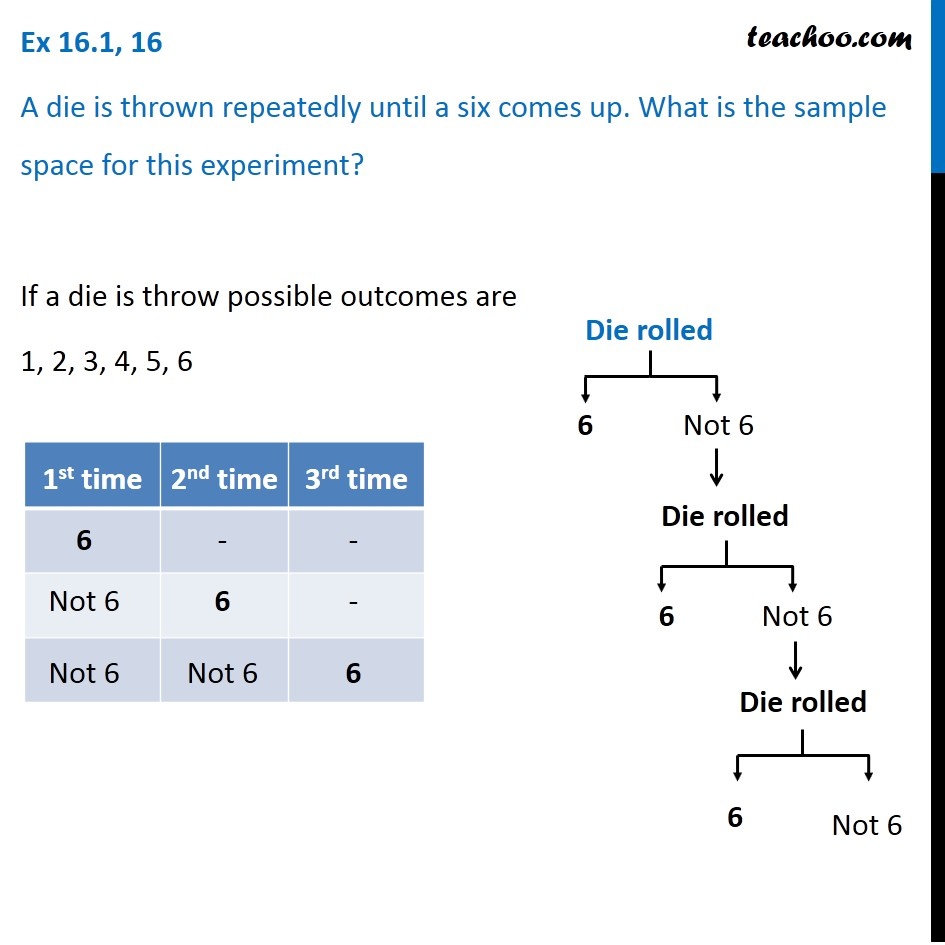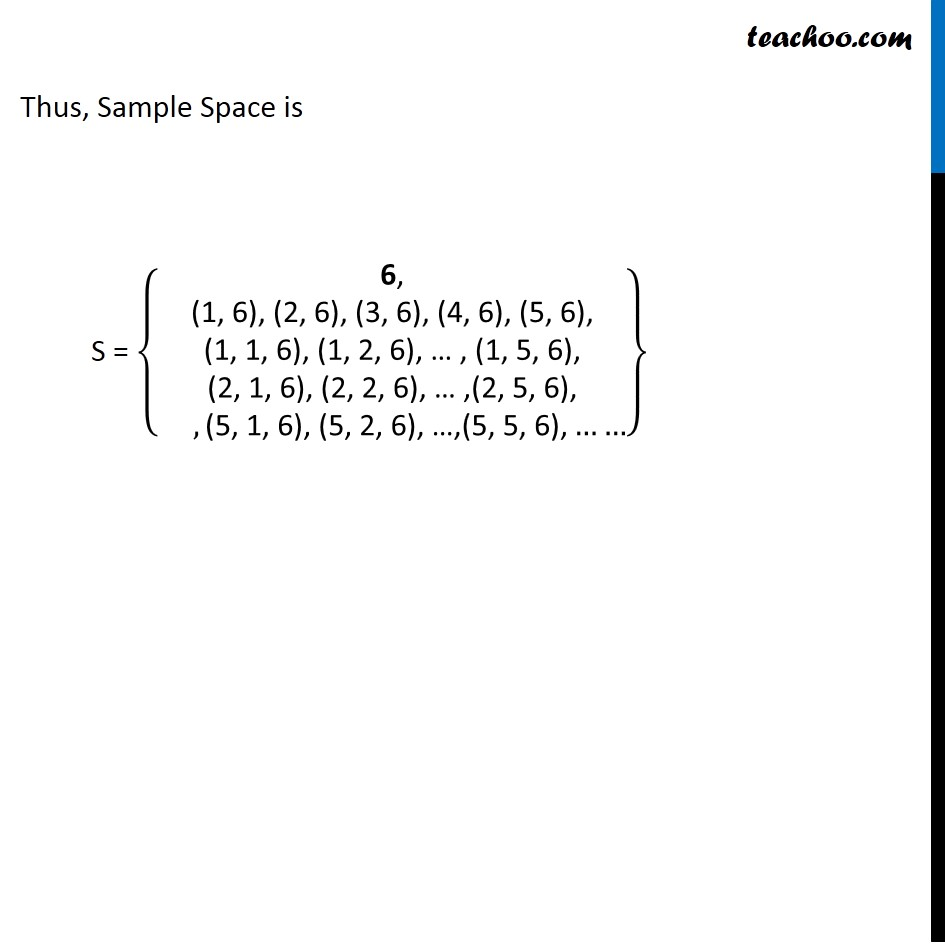Sample Space

Chapter 14 Class 11 Probability
Serial order wiseLearn in your speed, with individual attention - Teachoo Maths 1-on-1 Class

### Transcript

Question 16 A die is thrown repeatedly until a six comes up. What is the sample space for this experiment? If a die is throw possible outcomes are 1, 2, 3, 4, 5, 6 S = {█("6, (1, 6), (2, 6), (3, 6), (4, 6), (5, 6)," @" (1, 1, 6), (1, 2, 6), … , (1, 5, 6), " @"(2, 1, 6), (2, 2, 6), … ,(2, 5, 6)," @" " ,"(5, 1, 6), (5, 2, 6), …,(5, 5, 6)," ……)} Thus, Sample Space is S = {█("6," @"(1, 6), (2, 6), (3, 6), (4, 6), (5, 6)," @" (1, 1, 6), (1, 2, 6), … , (1, 5, 6), " @"(2, 1, 6), (2, 2, 6), … ,(2, 5, 6)," @" " ,"(5, 1, 6), (5, 2, 6), …,(5, 5, 6)," ……)}